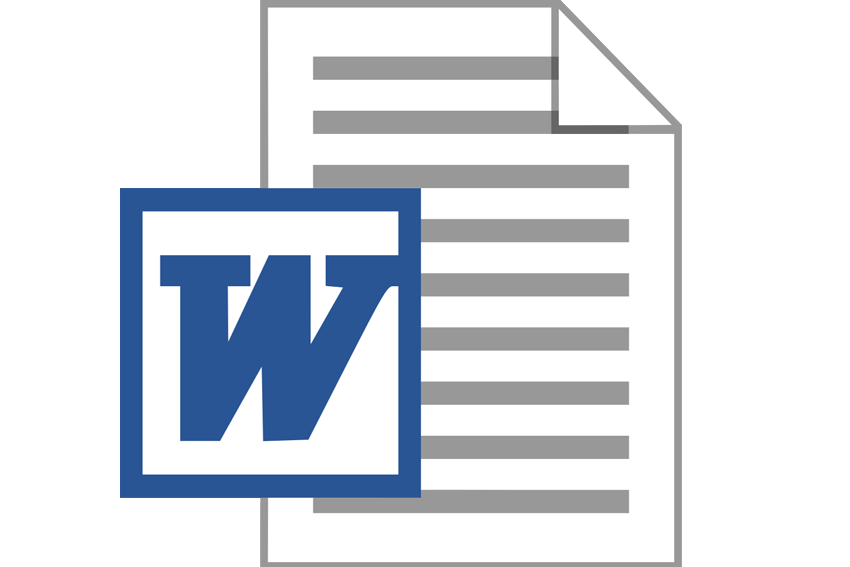We're Open
+44 7340 9595 39
+44 20 3239 6980

# Derive the ordinary least squares normal equations to estimate equation (1). Explain your answer.100% Pass and No Plagiarism Guaranteed

### Derive the ordinary least squares normal equations to estimate equation (1). Explain your answer.

Question 1

Identify the FALSE or INCORRECT statements in the following extract. Provide a brief explanation of your answers.

A researcher is interested in the relation between the yield on 20-yearBritish Government securities or gilts (denoted IG in the following Eviewsoutput) and the discount rate on 3-month sterling Treasury bills (denoted ITB in the Eviews output), for the period April 2001 to July 2016. The monthly data is from www.bankofengland.co.uk.

Dependent Variable: IG

Method: Least Squares

Sample: 2001M04 2016M07

Included observations: 184

 Variable Coefficient Std. Error t-Statistic Prob. C 3.395172 0.070661 48.04854 0.0000 ITB 0.255711 0.022067 11.58781 0.0000
 R-squared 0.424556 Adjusted R-squared 0.421394 S.E. of regression 0.619406 Sum squared resid 69.8269 Log likelihood -171.944
 Mean dependent var 4.02004 S.D. dependent var 0.8143 Akaike info criterion 1.8907 Schwarz criterion 1.92565 Hannan-Quinn criter. 1.90486
 C330 Econometric Principles and Data Analysis Session One 2017 F-statistic 134.2774 Durbin-Watson stat 0.097931 Prob(F-statistic) 0.000000

The population regression equation is

IG3.395172 0.255711ITBt

where IGis the yield on gilts at time t, and ITBis the discount rate on Treasury bills at time t.

There appears to be a positive relationship between IGand ITB. The estimated slope coefficient is 0.255711. The mean value for ITBin the Eviews output is 4.020043.

To test the hypothesis that the slope coefficient is zero, we can use a t-test. It is a two- variable model estimated using monthly data, so the appropriate degrees of freedom for this test are 10. The calculated t-statistic of 11.58781 and Prob. value of 0.0000 indicate we would not reject the hypothesis that the slope coefficient is equal to zero at the 0.05 significance level. Approximately 42.46% of the variation in IGis not

explained by the model. A 95% confidence interval for the slope coefficient would not include zero. The total sum of squares is less than 69.82689. The model is linear in the parameters to be estimated.

Question 2

Consider the two-variable regression model for which the population regression equation can be written in the following form:

 Yi 1 2 Xi ui (1) where Yi and Xi are observable variables, 1 and 2 are unknown regression

coefficients, and uis an unobservable population disturbance term.

The corresponding ordinary least squares sample regression equation is given by:

 Y ˆ ˆ X i e (2) i 1 2 i

where ˆand ˆare the estimators of the constant and the slope parameter respectively, and eis the sample residual for the ith observation.

 (a) Specify the ordinary least squares estimation criterion. [5 marks]

(b)Derive the ordinary least squares normal equations to estimate

(c)Discuss the conditions under which the estimators ˆand ˆ2

 obtained from the equations derived in 2(b) are BLUE. [10 marks]C330 Econometric Principles and Data Analysis Session One 2017

Question 3

The tab-delimited text file C330_A1_S1_2017_Q3.txt contains weekly data on the stock price of Hasbro Inc., the US multinational toys, games and entertainment company (HAS), and the Standard & Poor’s 500 index (SP500) from 27 August 2012 to 22 August 2016, giving 209 observations (the share price and stock index are closing prices, in US dollars, source: uk.finance.yahoo.com). For reference, for observation 27/8/2012 HAS = 37.51 and SP500 = 1406.58. For 4/9/2012 HAS = 37.64 and SP500 = 1437.92. And for 22/8/2016 HAS = 80.72 and SP500 = 2182.64. Use a 5% significance level in your analysis.

(a)Obtain a scatter plot of the weekly log return on the Hasbro Inc. stock

ln HASln HAS1

 against the weekly log return on the S&P 500 index for the complete sample, and comment on the plot. [10 marks]

(b)Use Eviews to estimate the following model using ordinary least squares over the full sample, and submit your output as your answer:

ln HASln HAS1 1 2 ln SP500ln SP500ut

[10 marks]

(c)Comment on the estimated parameters, including the relationship between the variables and the size of the estimated coefficient ˆ.

[10 marks]

(d)(i) Test whether the parameter is significantly different from zero.

 (ii) Test whether the parameter 2 is less than 1. [10 marks in total]

(e)Explain what the value of Rmeans in the context of the estimation of this model. Use the F-test to test whether the

 calculated R2 you obtain in Part (b) is significantly different from zero.

100% Plagiarism Free & Custom Written,International House, 12 Constance Street, London, United Kingdom,
E16 2DQ

## STILL NOT CONVINCED?

View our samples written by our professional writers to let you comprehend how your work is going to look like. We have categorised this into 3 categories with a few different subject domains

View Our Samples

#### We offer a £ 2999If your assignment is plagiarised, we will give you £ 2999 in compensation

Oct,14,2021

Oct,14,2021

Oct,14,2021

### Details

• Title: Derive the ordinary least squares normal equations to estimate equation (1). Explain your answer.
• Price: £ 79
• Post Date: 2018-11-10T08:12:04+00:00
• Category: Assignment
• No Plagiarism Guarantee
• 100% Custom Written

### Customer ReviewsDerive the ordinary least squares normal equations to estimate equation (1). Explain your answer.
Reviews: 5

### A masterpiece of assignment by Izkiyah , written on 2020-03-12

The support team is quite friendly and gives you the best solutions. I literally came here crying and went smiling like an idiot. My HR assignment is written beautifully with all the specifications I wanted. This is the best platform to get helped in assignments for me. All the people are supportive. Keep it up you all!
Reviews: 5

### A masterpiece of assignment by Meredith, written on 2020-03-12

I was worried about the plagiarism ration in my dissertation. But thanks to my dedicated writer, I received 0% plagiarism in all the chapters. I owe my writer a million thanks..!